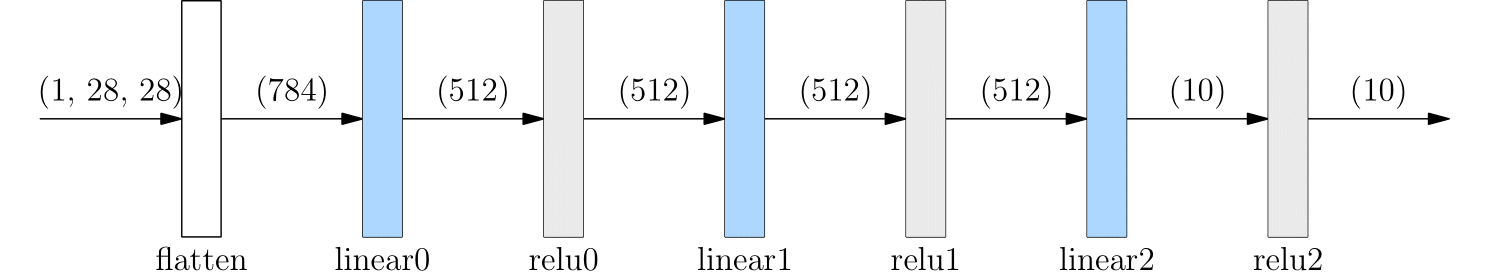# BUILD NEURAL NETWORK¶

The layers of a neural network can be built by API in namespace oneflow.nn, It provides common Module (such as oneflow.nn.Conv2d, oneflow.nn.ReLU). All Module classes inherit from oneflow.nn.Module, and many simple Module can form more complex Module. In this way, users can easily build and manage complex neural networks.

import oneflow as flow
import oneflow.nn as nn


## Define Module class¶

oneflow.nn provides common Module classes and we can use them easily. Or we can build a neural network by customizing the Module class on the basis. This method consists of three parts：

• Write a class that inherits from oneflow.nn.Module class
• Write the method of __init__ class, in which we construct the network structure
• Write the method of forward class, which calculates on the basis of the input of Module
class NeuralNetwork(nn.Module):
def __init__(self):
super(NeuralNetwork, self).__init__()
self.flatten = nn.Flatten()
self.linear_relu_stack = nn.Sequential(
nn.Linear(28*28, 512),
nn.ReLU(),
nn.Linear(512, 512),
nn.ReLU(),
nn.Linear(512, 10),
nn.ReLU()
)

def forward(self, x):
x = self.flatten(x)
logits = self.linear_relu_stack(x)
return logits
net = NeuralNetwork()
print(net)


The above code will output the structure of the NeuralNetwork network：

NeuralNetwork(
(flatten): Flatten(start_dim=1, end_dim=-1)
(linear_relu_stack): Sequential(
(0): Linear(in_features=784, out_features=512, bias=True)
(1): ReLU()
(2): Linear(in_features=512, out_features=512, bias=True)
(3): ReLU()
(4): Linear(in_features=512, out_features=10, bias=True)
(5): ReLU()
)
)


Then, call net (notice：It is not recommended to explicitly call forward):

X = flow.ones(1, 28, 28)
logits = net(X)
pred_probab = nn.Softmax(dim=1)(logits)
y_pred = pred_probab.argmax(1)
print(f"Predicted class: {y_pred}")


You will get output similar to the following:

Predicted class: tensor(, dtype=oneflow.int32)


The above process of data input, network calculation and the output of reasoning is shown in the figure below.## flow.nn.functional¶

In addition to oneflow.nn, oneflow.nn.functional namespace also provides many API. It overlaps with oneflow.nn to some extent. For example, nn.functional.relu and nn.ReLU both can be used for activation in neural network.

The main differences between them are:

• The API under nn is a class. It needs to be instantiated before being called; The API under nn.functional is a function. It is called directly.
• The API under nn manages network parameters automatically；But for the function under NN. Functional, we need to define our own parameters and manually pass them in each call.

In fact, most of the Module provided by OneFlow is the result of encapsulating the methods under nn.functional. nn.functional can manage the network more finely.

The following example uses the methods in nn.functional to build a Module FunctionalNeuralNetwork equivalent to the NeuralNetwork class above. Readers can appreciate the similarities and differences between the two:

class FunctionalNeuralNetwork(nn.Module):

def __init__(self):
super(FunctionalNeuralNetwork, self).__init__()

self.weight1 = nn.Parameter(flow.randn(28*28, 512))
self.bias1 = nn.Parameter(flow.randn(512))

self.weight2 = nn.Parameter(flow.randn(512, 512))
self.bias2 = nn.Parameter(flow.randn(512))

self.weight3 = nn.Parameter(flow.randn(512, 10))
self.bias3 = nn.Parameter(flow.randn(10))

def forward(self, x):
x = x.reshape(1, 28*28)
out = flow.matmul(x, self.weight1)
out = out + self.bias1
out = nn.functional.relu(out)

out = flow.matmul(out, self.weight2)
out = out + self.bias2
out = nn.functional.relu(out)

out = flow.matmul(out, self.weight3)
out = out + self.bias3
out = nn.functional.relu(out)

return out

net = FunctionalNeuralNetwork()
X = flow.ones(1, 28, 28)
logits = net(X)
pred_probab = nn.Softmax(dim=1)(logits)
y_pred = pred_probab.argmax(1)
print(f"Predicted class: {y_pred}")


## Module container¶

Comparing the similarities and differences between the NeuralNetwork and FunctionalNeuralNetwork,we can find that nn.Sequential plays an important role in simplifying the code.

nn.Sequential is a special container. Any class inherited from nn.Module can be placed in it.

Its specialty is that when Sequential propagates forward, Sequential automatically "concatenates" the layers contained in the container. Specifically, the output of the previous layer will be automatically transferred as the input of the next layer according to the sequence of Sequential added to each layer until the output of the last layer of the whole Moudle is obtained.

The following is an example of building a network without Sequential (not recommended):

class MyModel(nn.Module):
def __init__(self):
super(MyModel, self).__init__()
self.conv1 = nn.Conv2d(1,20,5)
self.relu1 = nn.ReLU()
self.conv2 = nn.Conv2d(20,64,5)
self.relu2 = nn.ReLU()

def forward(self, x):
out = self.conv1(x)
out = self.relu1(out)
out = self.conv2(out)
out = self.relu2(out)
return out


If sequential is used, it looks like this, which will be more concise.

class MySeqModel(nn.Module):
def __init__(self):
super(MySeqModel, self).__init__()
self.seq = nn.Sequential(
nn.Conv2d(1,20,5),
nn.ReLU(),
nn.Conv2d(20,64,5),
nn.ReLU()
)

def forward(self, x):
return self.seq(x)


Besides Sequential, there are nn.ModuleList and nn.ModuleDict. They can automatically register parameters to the whole network. But their other behavior is similar to Python list and Python dict, which are just simple containers and do not automatically propagate forward. You need manually traverse to complete the calculation of each layer.